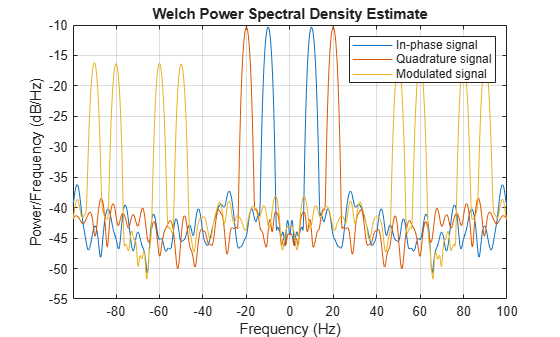# modulate

Modulation for communications simulation

## Syntax

``y = modulate(x,fc,fs)``
``[y,t] = modulate(x,fc,fs)``
``[___] = modulate(x,fc,fs,method)``
``[___] = modulate(x,fc,fs,method,opt)``

## Description

````y = modulate(x,fc,fs)` modulates the real message signal `x` with a carrier frequency `fc` and sample rate `fs`. If `x` is a matrix, the modulated signal is computed independently for each column and stored in the corresponding column of `y`.```
````[y,t] = modulate(x,fc,fs)` also returns the internal time vector `t`.```

example

````[___] = modulate(x,fc,fs,method)` modulates the real message signal using the modulation technique specified by `method`. You can use these inputs with either of the previous output syntaxes.```

example

````[___] = modulate(x,fc,fs,method,opt)` uses the additional options specified in `opt` for some modulation methods.```

## Examples

collapse all

Generate a 10 Hz sinusoidal signal sampled at a rate of 200 Hz for 1 second. Embed the sinusoid in white Gaussian noise of variance 0.01.

```fs = 200; t = 0:1/fs:1; x = sin(2*pi*10*t) + randn(size(t))/10;```

Single-sideband amplitude modulate the signal with a carrier frequency of 50 Hz. Compute and display the new Welch's power spectral density estimates.

```y = modulate(x,50,fs,'amssb'); pwelch([x;y]',hamming(100),80,1024,fs,'centered')```Generate two sinusoidal signals frequencies of 10 Hz and 20 Hz, sampled at a rate of 200 Hz for 1 second. Embed the sinusoids in white Gaussian noise of variance 0.01.

```fs = 200; t = 0:1/fs:1; i = sin(2*pi*10*t) + randn(size(t))/10; q = sin(2*pi*20*t) + randn(size(t))/10;```

Create a quadrature amplitude modulated signal from signals `i` and `q` using a carrier frequency of 70 Hz. Compute the Welch power spectral density estimates of the original and modulated sequences. Use a 100-sample Hamming window with 80 samples of overlap. Specify an FFT length of 1024.

```y = modulate(i,70,fs,'qam',q); pwelch([i;q;y]',hamming(100),80,1024,fs,'centered') legend('In-phase signal','Quadrature signal','Modulated signal')```## Input Arguments

collapse all

Message signal, specified as a real vector or matrix.

Example: `sin(2*pi*25*[0:(1/200):1])`

Carrier frequency used to modulate the message signal, specified as a real positive scalar.

Sample rate, specified as a real positive scalar.

Method of modulation used, specified as one of:

• `amdsb-sc` or `am` — Amplitude modulation, double sideband, suppressed carrier. Multiplies `x` by a sinusoid of frequency `fc`.

```y = x.*cos(2*pi*fc*t) ```
• `amdsb-tc` — Amplitude modulation, double sideband, transmitted carrier. Subtracts scalar `opt` from `x` and multiplies the result by a sinusoid of frequency `fc`.

```y = (x-opt).*cos(2*pi*fc*t) ```

If you do not specify the `opt` parameter, `modulate` uses a default of `min(min(x))` so that the message signal `(x-opt)` is entirely nonnegative and has a minimum value of 0.

• `amssb` — Amplitude modulation, single sideband. Multiplies `x` by a sinusoid of frequency `fc` and adds the result to the Hilbert transform of `x` multiplied by a phase-shifted sinusoid of frequency `fc`.

```y = x.*cos(2*pi*fc*t)+imag(hilbert(x)).*sin(2*pi*fc*t) ```

This effectively removes the upper sideband.

• `fm` — Frequency modulation. Creates a sinusoid with instantaneous frequency that varies with the message signal `x`.

`y = cos(2*pi*fc*t + opt*cumsum(x))`

`cumsum` is a rectangular approximation of the integral of `x`. `modulate` uses `opt` as the constant of frequency modulation. If you do not specify the `opt` parameter, `modulate` uses a default of `opt = (fc/fs)*2*pi/(max(max(x)))` so the maximum frequency excursion from `fc` is `fc` Hz.

• `pm` — Phase modulation. Creates a sinusoid of frequency `fc` whose phase varies with the message signal `x`.

`y = cos(2*pi*fc*t + opt*x)`

`modulate` uses `opt` as the constant of phase modulation. If you do not specify the `opt` parameter, `modulate` uses a default of `opt = pi/(max(max(x)))` so the maximum phase excursion is π radians.

• `pwm` — Pulse-width modulation. Creates a pulse-width modulated signal from the pulse widths in `x`. The elements of `x` must be between 0 and 1, specifying the width of each pulse in fractions of a period. The pulses start at the beginning of each period, that is, they are left justified. `modulate(x,fc,fs,'pwm','centered')` yields pulses centered at the beginning of each period. The length of `y` is `length(x)*fs/fc`.

• `ppm` — Pulse-position modulation. Creates a pulse-position modulated signal from the pulse positions in `x`. The elements of `x` must be between 0 and 1, specifying the left edge of each pulse in fractions of a period. `opt` is a scalar between 0 and 1 that specifies the length of each pulse in fractions of a period. The default for `opt` is `0.1`. The length of `y` is `length(x)*fs/fc`.

• `qam`— Quadrature amplitude modulation. Creates a quadrature amplitude modulated signal from signals `x` and `opt`.

`y = x.*cos(2*pi*fc*t) + opt.*sin(2*pi*fc*t)`

The input argument `opt` must be the same size as `x`.

Optional input, specified for some methods. Refer to `method` for more details on how to use `opt`.

## Output Arguments

collapse all

Modulated message signal, returned as a real vector or matrix. Except for the methods `pwm` and `ppm`, `y` is the same size as `x`.

Internal time array used by `modulate` in its computations, specified as a real vector.